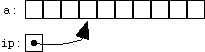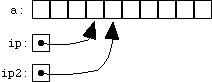## 10.2 Pointers and Arrays; Pointer Arithmetic

[This section corresponds to K&R Sec. 5.3]

Pointers do not have to point to single variables. They can also point at the cells of an array. For example, we can write

```	int *ip;
int a;
ip = &a;
```
and we would end up with ip pointing at the fourth cell of the array a (remember, arrays are 0-based, so a is the first cell). We could illustrate the situation like this:We'd use this ip just like the one in the previous section: *ip gives us what ip points to, which in this case will be the value in a.

Once we have a pointer pointing into an array, we can start doing pointer arithmetic. Given that ip is a pointer to a, we can add 1 to ip:

```	ip + 1
```
What does it mean to add one to a pointer? In C, it gives a pointer to the cell one farther on, which in this case is a. To make this clear, let's assign this new pointer to another pointer variable:
```	ip2 = ip + 1;
```
Now the picture looks like this:If we now do
```	*ip2 = 4;
```
we've set a to 4. But it's not necessary to assign a new pointer value to a pointer variable in order to use it; we could also compute a new pointer value and use it immediately:
```	*(ip + 1) = 5;
```
In this last example, we've changed a again, setting it to 5. The parentheses are needed because the unary ``contents of'' operator * has higher precedence (i.e., binds more tightly than) the addition operator. If we wrote *ip + 1, without the parentheses, we'd be fetching the value pointed to by ip, and adding 1 to that value. The expression *(ip + 1), on the other hand, accesses the value one past the one pointed to by ip.

Given that we can add 1 to a pointer, it's not surprising that we can add and subtract other numbers as well. If ip still points to a, then

```	*(ip + 3) = 7;
```
sets a to 7, and
```	*(ip - 2) = 4;
```
sets a to 4.

Up above, we added 1 to ip and assigned the new pointer to ip2, but there's no reason we can't add one to a pointer, and change the same pointer:

```	ip = ip + 1;
```
Now ip points one past where it used to (to a, if we hadn't changed it in the meantime). The shortcuts we learned in a previous chapter all work for pointers, too: we could also increment a pointer using
```	ip += 1;
```
or
```	ip++;
```

Of course, pointers are not limited to ints. It's quite common to use pointers to other types, especially char. Here is the innards of the mystrcmp function we saw in a previous chapter, rewritten to use pointers. (mystrcmp, you may recall, compares two strings, character by character.)

```	char *p1 = &str1, *p2 = &str2;

while(1)
{
if(*p1 != *p2)
return *p1 - *p2;
if(*p1 == '\0' || *p2 == '\0')
return 0;
p1++;
p2++;
}
```

The autoincrement operator ++ (like its companion, --) makes it easy to do two things at once. We've seen idioms like a[i++] which accesses a[i] and simultaneously increments i, leaving it referencing the next cell of the array a. We can do the same thing with pointers: an expression like *ip++ lets us access what ip points to, while simultaneously incrementing ip so that it points to the next element. The preincrement form works, too: *++ip increments ip, then accesses what it points to. Similarly, we can use notations like *ip-- and *--ip.

As another example, here is the strcpy (string copy) loop from a previous chapter, rewritten to use pointers:

```	char *dp = &dest, *sp = &src;
while(*sp != '\0')
*dp++ = *sp++;
*dp = '\0';
```

(One question that comes up is whether the expression *p++ increments p or what it points to. The answer is that it increments p. To increment what p points to, you can use (*p)++.)

When you're doing pointer arithmetic, you have to remember how big the array the pointer points into is, so that you don't ever point outside it. If the array a has 10 elements, you can't access a or a[-1] or even a (remember, the valid subscripts for a 10-element array run from 0 to 9). Similarly, if a has 10 elements and ip points to a, you can't compute or access ip + 10 or ip - 5. (There is one special case: you can, in this case, compute, but not access, a pointer to the nonexistent element just beyond the end of the array, which in this case is &a. This becomes useful when you're doing pointer comparisons, which we'll look at next.)

Read sequentially: prev next up top

This page by Steve Summit // Copyright 1995, 1996 // mail feedback